# Venn Predictor

Venn Predictor (or Venn Machine) is a multiprobability classification system. The term multiprobability means that we announce several probability distributions for the new label rather than a single one.

Venn Predictor divides the old examples into categories, puts the current examples into one of the categories, and then uses the frequencies of labels in the chosen category as probabilities for the current object's label.

We observe a sequence of examples, where each example is a pair (object, label). Objects are drawn from a measurable space called the object space,, and labels are drawn from a measurable space called the label space,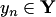. Their cartesian product,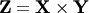is called the example space.

Venn Predictor deals with the case of classification, i.e., the case when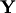is finite. Let us consider a general multiprobability prediction protocol. At each step of the protocol Reality announces, Predictor announces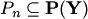(where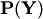is the set of all probability distributions on), and then Reality announces.

To define Venn Predictor formally, we need to introduce the concept of taxonomy. This is a sequence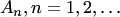, where each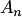is a measurable finite partition of the set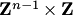. We write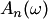for the element of the partitionthat contains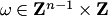. Venn Predictor can be defined for every taxonomy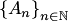. Let us consider the case when we have observed examples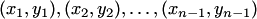and a new object,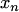. We denote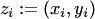. Let us consider the case when the current object has label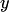and let us write (for now)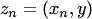. At each step of the protocol Venn Predictor divides the examples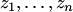into categories assigning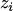and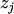to the same category if and only if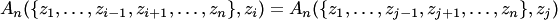, where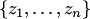denotes a multiset (a bag), i.e., a set of elements where each element has a multiplicity, i.e., a natural number indicating how many memberships it has in the multiset.

The category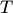containingis nonempty (it contains at least this one element). Let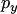be the empirical probability distribution on the labels in this category: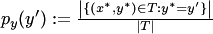; this is a probability distribution on. Venn Predictor determined by taxonomyis the multiprobability predictor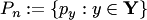. The setconsists of between one and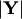distinct probability distributions on.

There are many Venn Predictors, one for each taxonomy. Some of them perform better then the others on particular datasets.